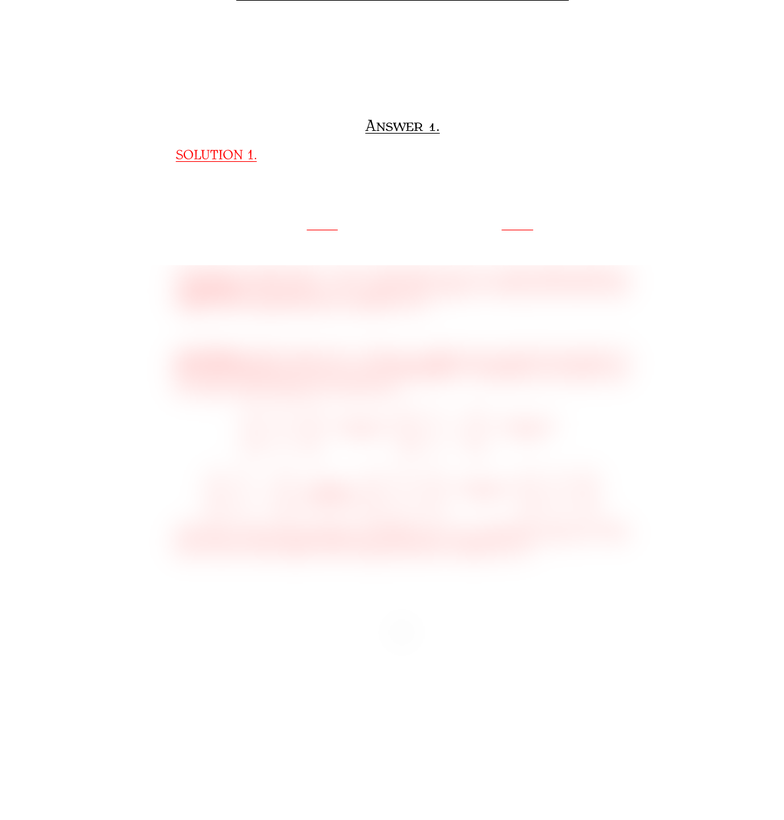Study Guides (400,000)
US (230,000)
BC (3,000)
MATH (90)
All (80)
Quiz

# Quiz3mt210Sample1AnsExam

This preview shows half of the first page. to view the full 1 pages of the document.MT Q  S 
İ S. Y
F , 
Surname, Name:
Q . §. T M E AX=B
Let u=
1
1
1
and A=
1 0 1
1 1 0
0 1 1
. Give two solutions answering the
following question:
Is uin the subset of R3spanned by the columns of A?
A .
SOLUTION 1. The vector uis in the subset spanned by the columns of Aif
and only if the system Ax=uhave a solution. Therefore, we need to decide
if Ax=uis consistent or not.
1011
1 1 0 1
0111
(1)R1+R2R2
//
1 0 1 1
0 1 1 2
0 1 1 1
(1)R2+R3R3
//
1 0 1 1
0 1 1 2
0 0 2 3
Note that we don’t have a row of the form [0 0 0 1]. The system above is
consistent by Theorem 2. As a result, the answer is "YES, uis in the in the
subset of R3spanned by the columns of A."
SOLUTION 2. The system Ax=bhave a solution for every bif and only if A
has a pivot position in every row by THEOREM 4. Therefore, we need to see
if Ahas a pivot position in every row:
1 0 1
1 1 0
0 1 1
(1)R1+R2R2
//
1 0 1
0 1 1
0 1 1
(1)R2+R3R3
//
1 0 1
0 1 1
0 0 2
(1/2)R3R3
(1)R3+R2R2
//
101
010
001
(1)R3+R1R1
//
100
010
001
Note that Ahas pivot positions in EVERY row. As a result, the answer is "YES,
uis in the in the subset of R3spanned by the columns of A."
1